## Cells use the hydrolysis of adenosine triphosphate, abbreviated as ATP, as a source of energy. Symbolically, this reaction can be written as

Question

Cells use the hydrolysis of adenosine triphosphate, abbreviated as ATP, as a source of energy. Symbolically, this reaction can be written asATP(aq)+H2O(l)⟶ADP(aq)+H2PO−4 (aq)where ADP represents adenosine diphosphate. For this reaction, ΔG∘=−30.5kJ/mol.a. Calculate K at 25∘C .b. If all the free energy from the metabolism of glucoseC6H12O6(s)+6O2(g)⟶6CO2(g)+6H2O(l)goes into forming ATP from ADP, how many ATP molecules can be produced for every molecule of glucose?

in progress 0
6 months 2021-08-27T22:52:58+00:00 1 Answers 0 views 0

Step-by-step explanation:

From the given information:

ΔG° = -30.5 kJ/mol

By applying the following equation to calculate the value of K.

ΔG° =-RT㏑K

making ㏑ K  the subject of the formula: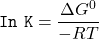where;

Temperature at 25° C = (25 + 273)K

= 298K

R = 8.3145 J/mol.K (gas cosntant)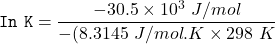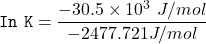㏑K = 12.309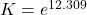K = 221682.17

K = 2.22 × 10⁵

b) The reaction for the metabolism of glucose is given as: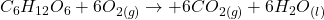From the above expression, let calculate the Gibbs free energy by using the formula: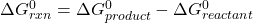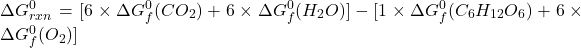At standard conditions;

The values of corresponding compounds are substituted into the equation above:

Thus,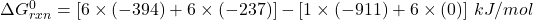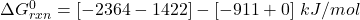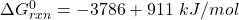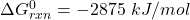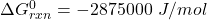Now, the no of ATP molecules generated =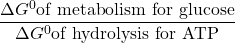= (-2875000 J/mol ) / -30500 J/mol

= 94.26

≅ 94 ATP molecules generated